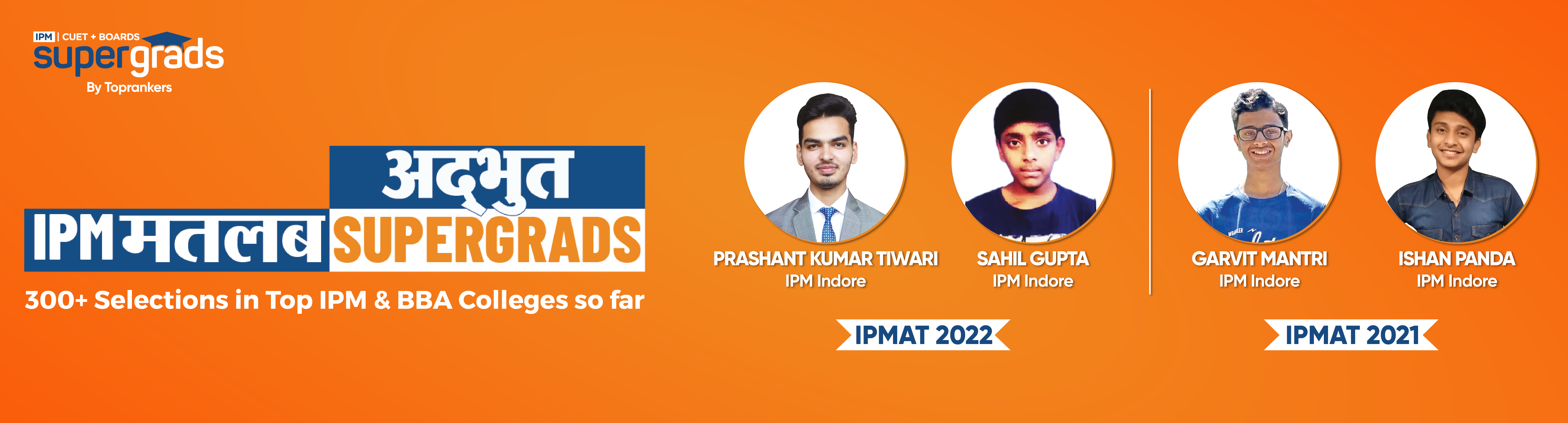• Home
• Quick Tricks to Solv...

# Quick Tricks to Solve Multiplication Division Questions in IPMAT 2024

Author : Tanya Kaushal

Updated On : July 28, 2023

SHARE

What to Expect from this Article? -  When appearing for competitive exams with aptitude as the main subject it is very obvious to be concerned about  solving questions in time.

If you are an aspirant for the IPMAT exam but worried about how to perform calculations in less time, you are at the right place.

Learn the different techniques for solving multiplication and division tricks for competitive exams. Solve questions faster by multiplication short tricks to save your time and attempt all the questions.

This post shall guide you through short tips and tricks for solving multiplication and division questions in the IPMAT exam.

Why Learn Quick Multiplication and Division Tricks?

The majority topics from Quantitative section of IPMAT require the basic multiplication, and division. Mastering the shortcuts of multiplication, and division for IPMAT will give you extra time for other questions. Mainly, the topics including Number System, Averages & Percentages, Roots, Indices, Surds, Simple & Compound Interest, Profit & Loss, Algebraic Formulae, Linear & Quadratic Equations, Ratio & Proportion, Partnership, Mixtures & Alligations, Time, Speed & Distance, Work Related Problems, and Pipes & Cisterns require faster calculation technique of multiply and divide.

## Short Tricks to Attempt Multiplication Division Questions in IPMAT 2024

Learning simple tricks will help manage your time in the final exam and help you solve the questions with utmost accuracy. You can learn tips and tricks to improve your efficiency and accuracy within the stipulated time by learning simple tricks to attempt questions in the IPMAT Quant section.

Note: The quantitative tricks differ in length depending on the mathematical operation and the numbers involved. If you can calculate the problems in seconds by using short tricks, scoring the marks in the exam is easy.

Now, let us understand how to multiply and divide numbers using simple tricks from the post below.

### How to Multiply any Number by 5?

Without knowing the trick, many of you might waste your time multiplying a particular number with 5.

Generally, 5 is expressed as, 10/2.

Whenever you convert 5 from 10/2, the calculation becomes easy.

For Example:

If you want to multiply 72 X 5. Replace 5 by 10/2

Then, it will become 72 X 10/2

Divide the  72/2 , which is 36

Finally, multiply 36 with 10, (36X10). The final answer is 360

### How to Multiply any Number by 11?

Knowing the short trick to multiply any number by 11 will help you solve the problem in less than 5 seconds.

Suppose if you want to multiply PX11

• The last digit of the answer is the last digit of P
• The middle digit of the answer is the last digit of the sum of all digits of P
• The first digit of the answer is the first digit of P, and the carry (if any)

For Example:

Multiply 58X11 by using the trick mentioned above.

The last digit of the answer is the last digit of P. Here, P is 58, which means 8 is the last digit.

The middle digit of the answer is the last digit of the sum of all numbers of P: 5+8=13 i.e., 3

The first digit of the answer is the first digit of P plus they carry (if any): 5+1=6

Hence, the final answer for 58X11 is 638

### How to Multiply any Number by 15?

Consider multiplying 56 by 15 using the simple technique explained below:

Add a zero to the first number i.e., for 56. It becomes 560

Divide that number by 2. then we get 560/2=280

Add a resultant number to the consequent of the first number after adding zero i.e., 280+560 = 840

Hence, the final answer is 840

Read more: Important formulas for IPMAT Quant section

### How to Multiply any Number by 9?

Remember, multiplying any number by 9 is just multiplying as (10-1). You can follow the trick given below while multiplying any number by 9.

For Example:

Multiply 68 by 9, which means 68X9

Consider multiplying 9 as (10-1) i.e. 68X(10-1)

It becomes 680-68=612

Here, the final answer is 612## Important Rules for Solving Division Questions in IPMAT 2024

As per the previous year's trends, the difficulty level of questions asked in the IPMAT exam varies yearly. Therefore, learning some essential rules will help you solve any question in a matter of seconds.

The following are some important rules that you need to focus on while solving multiplication and division questions.

For Example:

560/2=280

### 2. If the sum of all numbers is divisible by 3, then the number is divisible by 3.

For Example:

Consider 861 (8+6+1=15)

15 is divisible by 3. Therefore, 861 is also divisible by 3

### 3. If the end of two digits of the number is divisible by 4 or the end of two-digit is zer0, the number is divisible by 4.

Read more: Important Topics for IPMAT 2024 exam

For Example:

Consider 1600 divided by 4

### 4. If the number's last digit is 0 or 5, then the number is divisible by 5.

For Example:

225 divided by 5### 5. If all digits sum of a number is divisible by 9, the number is divisible by 9.

For Example:

Consider whether 9522 is divisible by 9 or not.

9522=9+5+2+2=18

18 is divisible by 9. Therefore, 9522 is also divisible by 9

For Example:

4790/10

=479

### How to Divide any Number by 5?

To divide any number by 5, replace 5 with 10/2

Let us take 144/5, then 144/(10/2)

Now its become 144X2/10 = 288/10

What are the shortcuts for Division?What is the short cut for multiplication?Is 1 month enough for IPMAT Maths Preparation?How can I improve my accuracy during the IPMAT Maths Preparation?How can I better for IPMAT Maths Section?Which is the best Study Material for IPMAT Quant Preparation?Do I need to take specific coaching for IPMAT Maths?